Previous: 6.1.1.2 Von Neumann Equation Up: 6.1 Quantum Mechanics in Next: 6.1.3 The Riccati and

## 6.1.2 Hydrodynamical Formulation

The hydrodynamic model, originally proposed by Madelung [Mad27], provides a classical picture for quantum dynamics, namely, that of the flow of an indestructible probability fluid.

Mathematically it consists in representing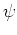using polar coordinates as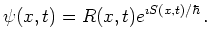(6.17)

Substituting this ansatz into the time-dependent Schrödinger equation with constant mass and separating into real and imaginary parts, gives two equations: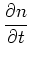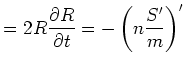(6.18)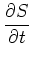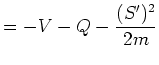(6.19)

where we introduced the quantum density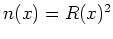and the so-called quantum potential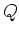: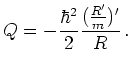(6.20)

In these formulas the prime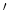denotes the one-dimensional space derivative.

The quantum potential arises from the kinetic energy of the Schrödinger equation and creates the self-field''. Alternatively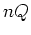can be seen as pressure in a hydrodynamical Navier-Stokes interpretation [Har66], which is related to Nelson's stochastic interpretation of quantum mechanics.

In the classical limit (e.g., WKB approximation [Kol00], [Sch69]) Equation 6.19 becomes the Hamilton-Jacobi equation with principal function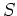.

With the identification of the velocity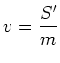(6.21)

and the flux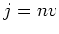(6.22)

the Equation 6.18 becomes the continuity equation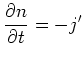(6.23)

and the second equation can be rewritten as an equation of motion (assuming constant mass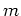)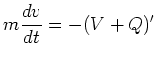(6.24)

where in the last equation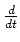denotes the Lagrangian (also called convective'') derivative [Bit00], [SRV89].

In the deBroglie-Bohm interpretation of quantum mechanics a particle has a sharp position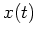at each time moving along a fluid trajectory determined by Equation 6.25 (with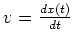). Evolution in the Bohm picture is sharp'', but the initial condition given by the initial location of the particle is a distribution. Tunneling is explained by lowering the barrier through the additional Bohm potential. This is a hidden variables theory which singles out position as a preferred variable. The same can be done for other observables, see [Vin00].

In the stationary case we have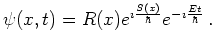(6.25)

This gives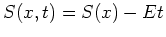and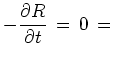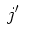(6.26)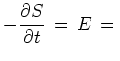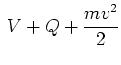(6.27)

which expresses conservation of particle number and of energy.

The hydrodynamical formulation can be extended to the mixed state case and was used for quantum mechanical simulation in [BM02], [LW99], [Dal03], [Bit00].

It is pointed out in [Wal94], that the hydrodynamical formulation needs an additional quantization constraint for equivalence with the standard'' Schrödinger equation.